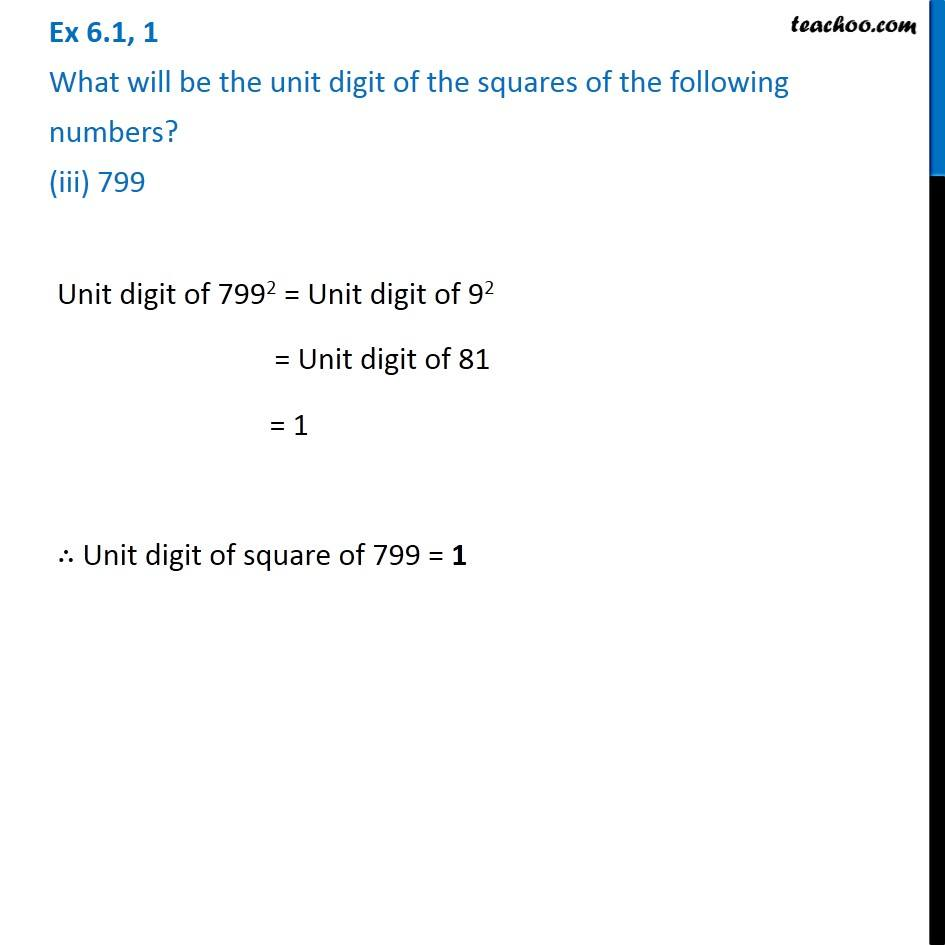Ex 6.1

Chapter 6 Class 8 Squares and Square Roots
Serial order wiseGet live Maths 1-on-1 Classs - Class 6 to 12

### Transcript

Ex 6.1, 1 What will be the unit digit of the squares of the following numbers? (iii) 799Unit digit of 7992 = Unit digit of 92 = Unit digit of 81 = 1 ∴ Unit digit of square of 799 = 1